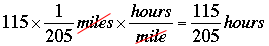SEARCH HOMEMath Central Quandaries & QueriesQuestion from tiana, a student: If a plane is taking off and its climbing at 380 feet per minute at 205 MPH, what is the altitude of the plane after it traveled 115 miles?Hi Tiana,

You are asked for the altitude and the altitude is increasing at 380 feet per minute so if you can find the number of minutes it takes to fly 115 miles you can multiply the number of minutes by 380 feet per minute to arrive at the altitude in feet. The plane travels 115 miles at 205 miles per hour so you should be able to use these numbers to determine how many hours that takes and then convert the hours to minutes.

Flying 115 miles at 205 miles per hour takesMultiply this number by 60 to convert the time to minutes and then use the fact that the altitude is increasing at 380 feet per minute to determine the altitude.

PennyMath Central is supported by the University of Regina and The Pacific Institute for the Mathematical Sciences.